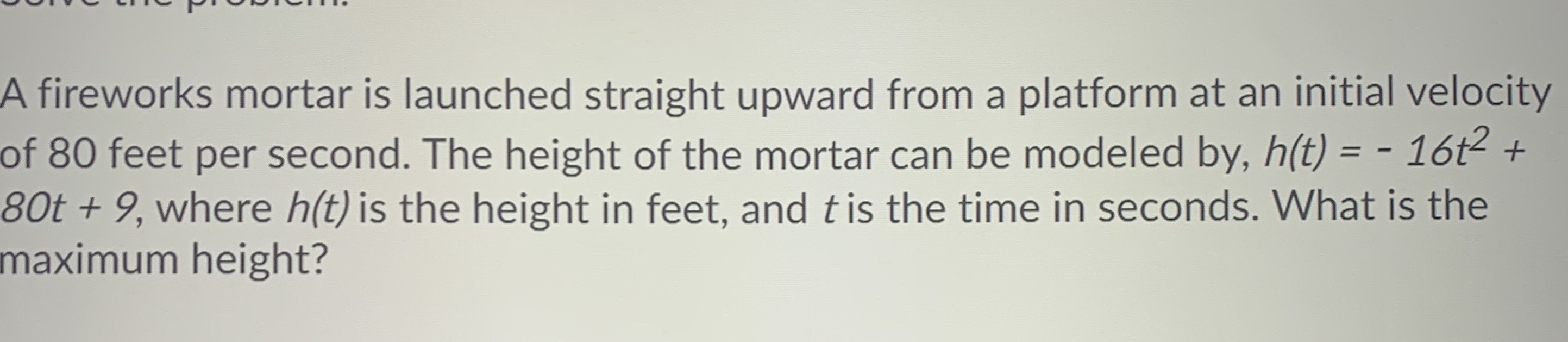### ¿Todavía tienes preguntas de matemáticas?

Pregunte a nuestros tutores expertos
Algebra
PreguntaA fireworks mortar is launched straight upward from a platform at an initial velocity of $$80$$ feet per second. The height of the mortar can be modeled by, $$h ( t ) = - 16 t ^ { 2 } +$$ $$80 t + 9 ,$$ where $$h ( t )$$ is the height in feet, and $$t$$ is the time in seconds. What is the maximum height?